Dodecahedron (adapted from Leonardo da Vinci)

This video is an adaptation of the drawing that Leonardo da Vinci made of the dodecahedron (duodecedron planus vacuus) for Luca Pacioli's book 'De Divina Proportione'.

Pacioli wrote about the dodecahedron (Spanish translation):

"El dodecaedro plano sólido o hueco tiene treinta líneas o lados iguales que forman en él sesenta ángulos superficiales, y tiene veinte ángulos sólidos y doce bases o superficies que lo contienen. Estas son todas pentagonales, de lados y ángulos iguales entre sí, como se deduce de su forma."
('La divina proporción' de Luca Pacioli, page 95,Spanish translation by Juan Calatrava, Editorial Akal, 4th edition, 2008)Leonardo da Vinci's drawing of the dodecahedron (duodecedron vacuus) for Luca Pacioli's book 'De divina proportione'. (There is an Spanish version, 'La divina proporción' Editorial Akal. Image used with permission of Editorial Akal).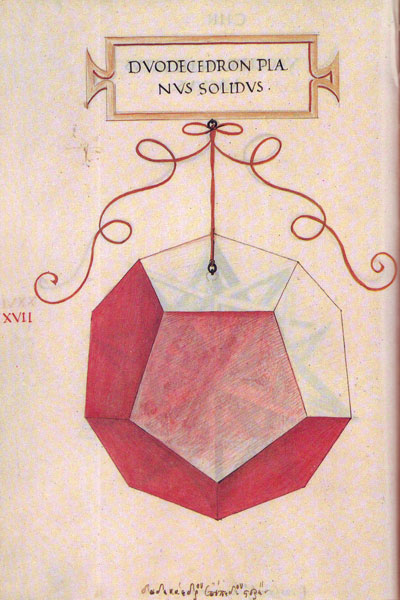Leonardo da Vinci's drawing of the dodecahedron (duodecedron solidus) for Luca Pacioli's book 'De divina proportione'. (There is an Spanish version, 'La divina proporción' Editorial Akal. Image used with permission of Editorial Akal).

If you play with the interactive application you can get images like these: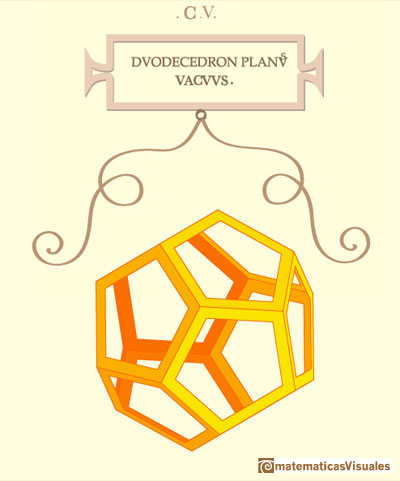REFERENCES

Luca Pacioli - La divina proporción - Ediciones Akal, 4th edition, 2004. Spanish edition of 'De divina proportione'. Translation by Juan Calatrava.
Leonardo da Vinci's Geometric Sketches Frank J. Swetz's article in MathDl, Loci:Convergence.
Leonardo da Vinci's Polyhedra George Hart's excellent website about polyhedra.Leonardo da Vinci made several drawings of polyhedra for Luca Pacioli's book 'De divina proportione'. Here we can see an adaptation of the cuboctahedron.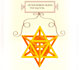Leonardo da Vinci made several drawings of polyhedra for Luca Pacioli's book 'De divina proportione'. Here we can see an adaptation of the stellated octahedron (stella octangula).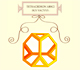Leonardo da Vinci made several drawings of polyhedra for Luca Pacioli's book 'De divina proportione'. Here we can see an adaptation of the truncated tetrahedron.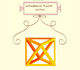Leonardo da Vinci made several drawings of polyhedra for Luca Pacioli's book 'De divina proportione'. Here we can see an adaptation of the octahedron.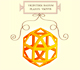Leonardo da Vinci made several drawings of polyhedra for Luca Pacioli's book 'De divina proportione'. Here we can see an adaptation of the rhombicuboctahedron.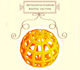Leonardo da Vinci made several drawings of polyhedra for Luca Pacioli's book 'De divina proportione'. Here we can see an adaptation of the Campanus' sphere.Some properties of this platonic solid and how it is related to the golden ratio. Constructing dodecahedra using different techniques.The first drawing of a plane net of a regular dodecahedron was published by Dürer in his book 'Underweysung der Messung' ('Four Books of Measurement'), published in 1525 .The diagonal of a regular pentagon are in golden ratio to its sides and the point of intersection of two diagonals of a regular pentagon are said to divide each other in the golden ratio or 'in extreme and mean ratio'.You can draw a regular pentagon given one of its sides constructing the golden ratio with ruler and compass.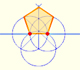In his book 'Underweysung der Messung' Durer draw a non-regular pentagon with ruler and a fixed compass. It is a simple construction and a very good approximation of a regular pentagon.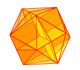The twelve vertices of an icosahedron lie in three golden rectangles. Then we can calculate the volume of an icosahedronA golden rectangle is made of an square and another golden rectangle.The golden spiral is a good approximation of an equiangular spiral.Two equiangular spirals contains all vertices of golden rectangles.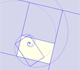A golden rectangle is made of an square an another golden rectangle. These rectangles are related through an dilative rotation.One eighth of a regular dodecahedon of edge 2 has the same volume as a dodecahedron of edge 1.The volume of a tetrahedron is one third of the prism that contains it.The first drawing of a plane net of a regular tetrahedron was published by Dürer in his book 'Underweysung der Messung' ('Four Books of Measurement'), published in 1525 .The volume of an octahedron is four times the volume of a tetrahedron. It is easy to calculate and then we can get the volume of a tetrahedron.The truncated octahedron is an Archimedean solid. It has 8 regular hexagonal faces and 6 square faces. Its volume can be calculated knowing the volume of an octahedron.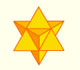The stellated octahedron was drawn by Leonardo for Luca Pacioli's book 'De Divina Proportione'. A hundred years later, Kepler named it stella octangula.A cuboctahedron is an Archimedean solid. It can be seen as made by cutting off the corners of a cube.A cuboctahedron is an Archimedean solid. It can be seen as made by cutting off the corners of an octahedron.These polyhedra pack together to fill space, forming a 3 dimensional space tessellation or tilling.The truncated tetrahedron is an Archimedean solid made by 4 triangles and 4 hexagons.When you truncate a cube you get a truncated cube and a cuboctahedron. If you truncate an octahedron you get a truncated octahedron and a cuboctahedron.